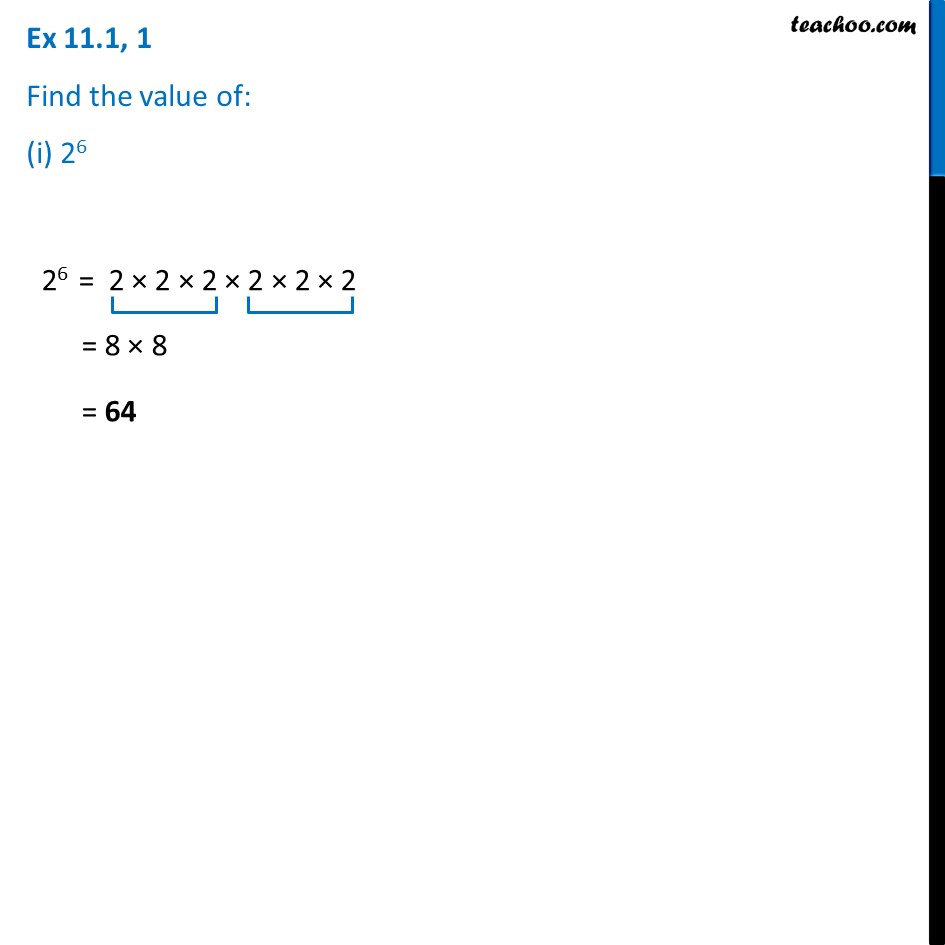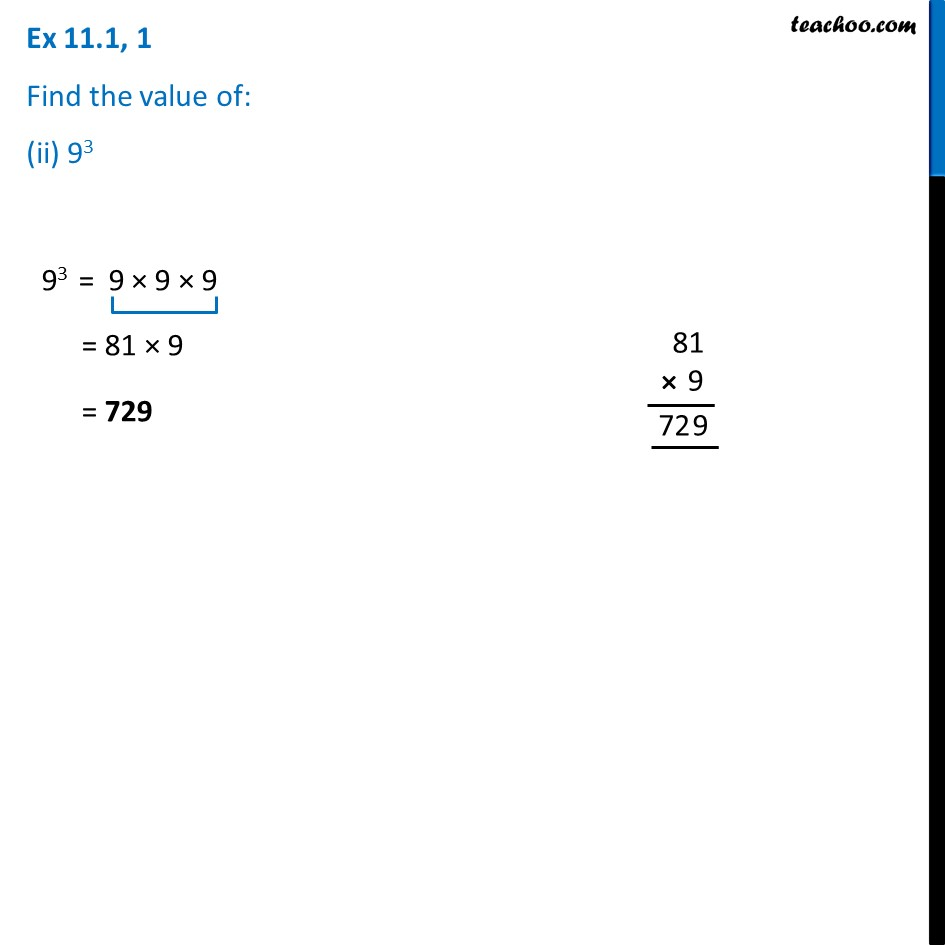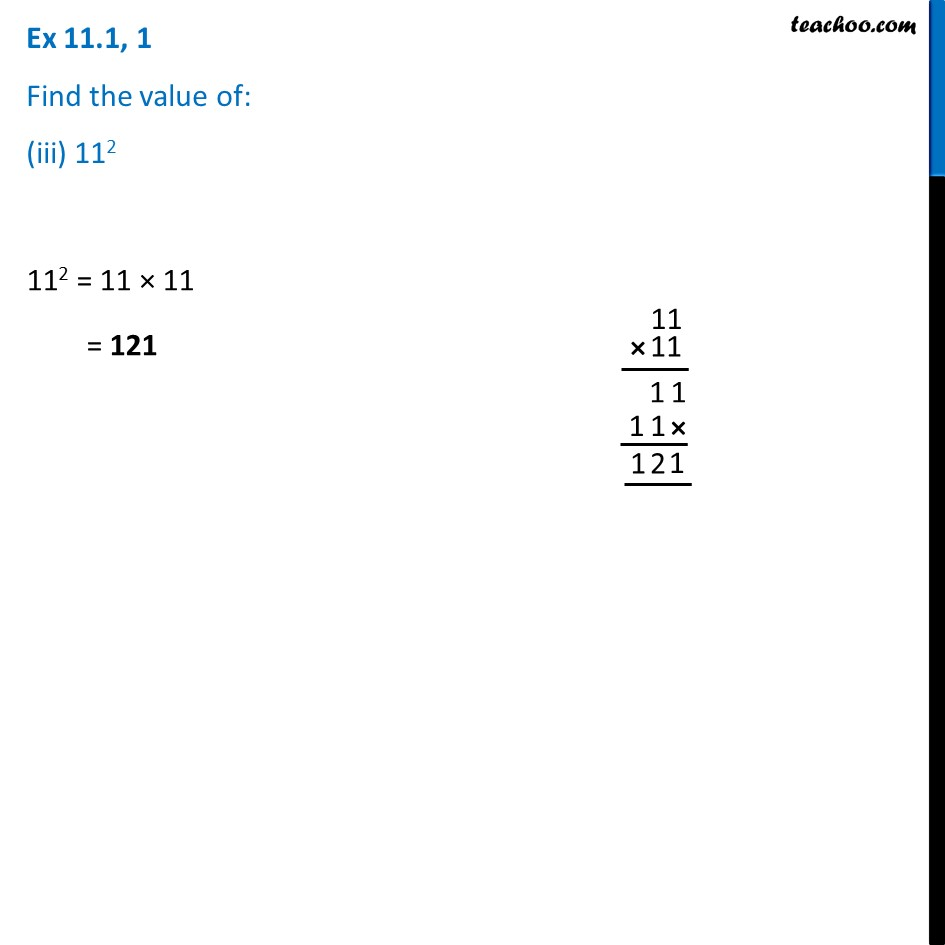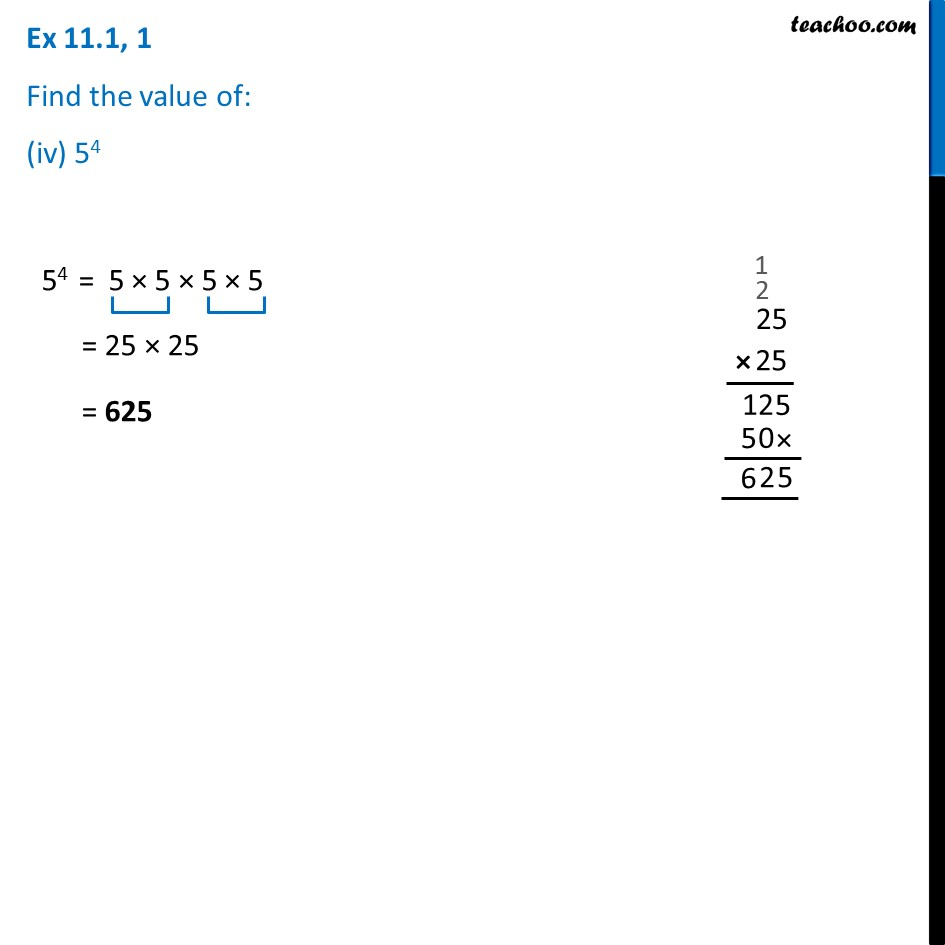Ex 11.1

Chapter 11 Class 7 Exponents and Powers
Serial order wiseLearn in your speed, with individual attention - Teachoo Maths 1-on-1 Class

### Transcript

Ex 11.1, 1 Find the value of: (i) 26 26 = 2 × 2 × 2 × 2 × 2 × 2 = 8 × 8 = 64 Ex 11.1, 1 Find the value of: (ii) 93 93 = 9 × 9 × 9 = 81 × 9 = 729 Ex 11.1, 1 Find the value of: (iii) 112 112 = 11 × 11 = 121 Ex 11.1, 1 Find the value of: (iv) 54 54 = 5 × 5 × 5 × 5 = 25 × 25 = 625